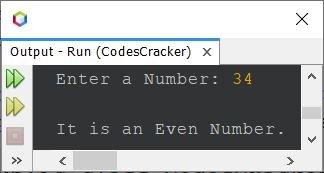# Java Program to Check Odd or Even Number

This article covers a program in Java that checks whether a number entered by user at run-time of the program, is an odd or an even number.

Note - A number that can be divided into two equal groups or parts, can be called as an even number. Whereas a number that can not be divided into two equal groups, can be called as an odd number.

## Check Odd or Even using if-else in Java

The question is, write a Java program to check odd or even number. The number must be received by user at run-time. The program given below is its answer:

```import java.util.Scanner;

public class CodesCracker
{
public static void main(String[] args)
{
int num;
Scanner scan = new Scanner(System.in);

System.out.print("Enter a Number: ");
num = scan.nextInt();

if(num%2==0)
{
System.out.println("\nIt is an Even Number.");
}
else
{
System.out.println("\nIt is an Odd Number.");
}
}
}```

The snapshot given below shows the sample run of above Java program on checking whether a given number is an odd or an even number, with user input 34The above program can also be written as:

```import java.util.Scanner;

public class CodesCracker
{
public static void main(String[] args)
{
Scanner scan = new Scanner(System.in);

System.out.print("Enter a Number: ");
int num = scan.nextInt();

if(num%2==0)
System.out.println("\n" +num+ " is an Even Number.");
else
System.out.println("\n" +num+ " is an Odd Number.");
}
}```

Here is its sample run with user input 7:## Check Odd or Even using Ternary Operator in Java

This program uses ternary operator (?:) to do the same job as done by previous program.

```import java.util.Scanner;

public class CodesCracker
{
public static void main(String[] args)
{
Scanner scan = new Scanner(System.in);

System.out.print("Enter a Number: ");
int num = scan.nextInt();

int res = (num%2==0) ? 0 : 1;

if(res==0)
System.out.println("\n" +num+ " is an Even Number.");
else
System.out.println("\n" +num+ " is an Odd Number.");
}
}```

The above program can also be created in this way:

```import java.util.Scanner;

public class CodesCracker
{
public static void main(String[] args)
{
Scanner scan = new Scanner(System.in);

System.out.print("Enter a Number: ");
int num = scan.nextInt();

String res = (num%2==0) ? "even" : "odd";

if(res.equals("even"))
System.out.println("\n" +num+ " is an Even Number.");
else
System.out.println("\n" +num+ " is an Odd Number.");
}
}```

## Check Odd or Even without using Modulus Operator in Java

This is the last program of this article, that checks for even or odd number. This program does not uses modulus (%) operator in any way.

```import java.util.Scanner;

public class CodesCracker
{
public static void main(String[] args)
{
Scanner scan = new Scanner(System.in);

System.out.print("Enter a Number: ");
int num = scan.nextInt();

float resReal = (float)num/2;
int resRound = num/2;

if((resReal*2)==(resRound*2))
System.out.println("\n" +num+ " is an Even Number.");
else
System.out.println("\n" +num+ " is an Odd Number.");
}
}```

#### Same Program in Other Languages

Java Online Test

« Previous Program Next Program »